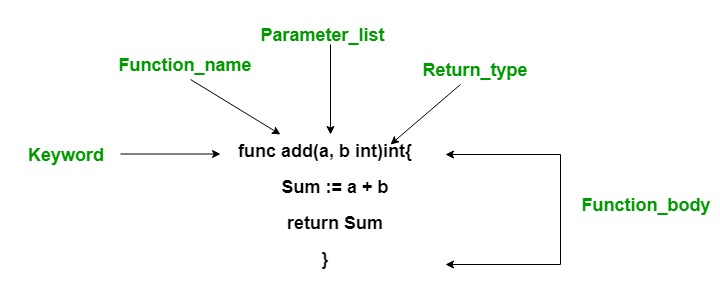# Functions in Go Language

• Difficulty Level : Basic
• Last Updated : 16 Oct, 2020

Functions are generally the block of codes or statements in a program that gives the user the ability to reuse the same code which ultimately saves the excessive use of memory, acts as a time saver and more importantly, provides better readability of the code. So basically, a function is a collection of statements that perform some specific task and return the result to the caller. A function can also perform some specific task without returning anything.

#### Function Declaration

Function declaration means a way to construct a function.
Syntax:

```func function_name(Parameter-list)(Return_type){
// function body.....
}

```

The declaration of the function contains:

• func: It is a keyword in Go language, which is used to create a function.
• function_name: It is the name of the function.
• Parameter-list: It contains the name and the type of the function parameters.
• Return_type: It is optional and it contain the types of the values that function returns. If you are using return_type in your function, then it is necessary to use a return statement in your function.#### Function Calling

Function Invocation or Function Calling is done when the user wants to execute the function. The function needs to be called for using its functionality. As shown in the below example, we have a function named as area() with two parameters. Now we call this function in the main function by using its name, i.e, area(12, 10) with two parameters.
Example:

## C

 `// Go program to illustrate the``// use of function``package main``import ``"fmt"` `// area() is used to find the``// area of the rectangle``// area() function two parameters,``// i.e, length and width``func area(length, width ``int``)``int``{``    ` `    ``Ar := length* width``    ``return` `Ar``}` `// Main function``func main() {``  ` `   ``// Display the area of the rectangle``   ``// with method calling``   ``fmt.Printf(``"Area of rectangle is : %d"``, area(12, 10))``}`

Output:

```Area of rectangle is : 120
```

#### Function Arguments

In Go language, the parameters passed to a function are called actual parameters, whereas the parameters received by a function are called formal parameters.
Note: By default Go language use call by value method to pass arguments in function.
Go language supports two ways to pass arguments to your function:

• Call by value: : In this parameter passing method, values of actual parameters are copied to function’s formal parameters and the two types of parameters are stored in different memory locations. So any changes made inside functions are not reflected in actual parameters of the caller.
Example:

## C

 `// Go program to illustrate the``// concept of the call by value``package main`` ` `import ``"fmt"`` ` `// function which swap values``func swap(a, b ``int``)``int``{` `    ``var o ``int``    ``o= a``    ``a=b``    ``b=o``   ` `   ``return` `o``}`` ` `// Main function``func main() {`` ``var p ``int` `= 10`` ``var q ``int` `= 20``  ``fmt.Printf(``"p = %d and q = %d"``, p, q)`` ` ` ``// call by values`` ``swap(p, q)``   ``fmt.Printf(``"\np = %d and q = %d"``,p, q)``}`

Output:

```p = 10 and q = 20
p = 10 and q = 20

```
•  Call by reference: Both the actual and formal parameters refer to the same locations, so any changes made inside the function are actually reflected in actual parameters of the caller.
Example:

## C

 `// Go program to illustrate the``// concept of the call by reference``package main`` ` `import ``"fmt"`` ` `// function which swap values``func swap(a, b *``int``)``int``{``    ``var o ``int``    ``o = *a``    ``*a = *b``    ``*b = o``    ` `   ``return` `o``}`` ` `// Main function``func main() {` ` ``var p ``int` `= 10`` ``var q ``int` `= 20`` ``fmt.Printf(``"p = %d and q = %d"``, p, q)`` ` ` ``// call by reference`` ``swap(&p, &q)``   ``fmt.Printf(``"\np = %d and q = %d"``,p, q)``}`

Output:

```p = 10 and q = 20
p = 20 and q = 10

```

My Personal Notes arrow_drop_up